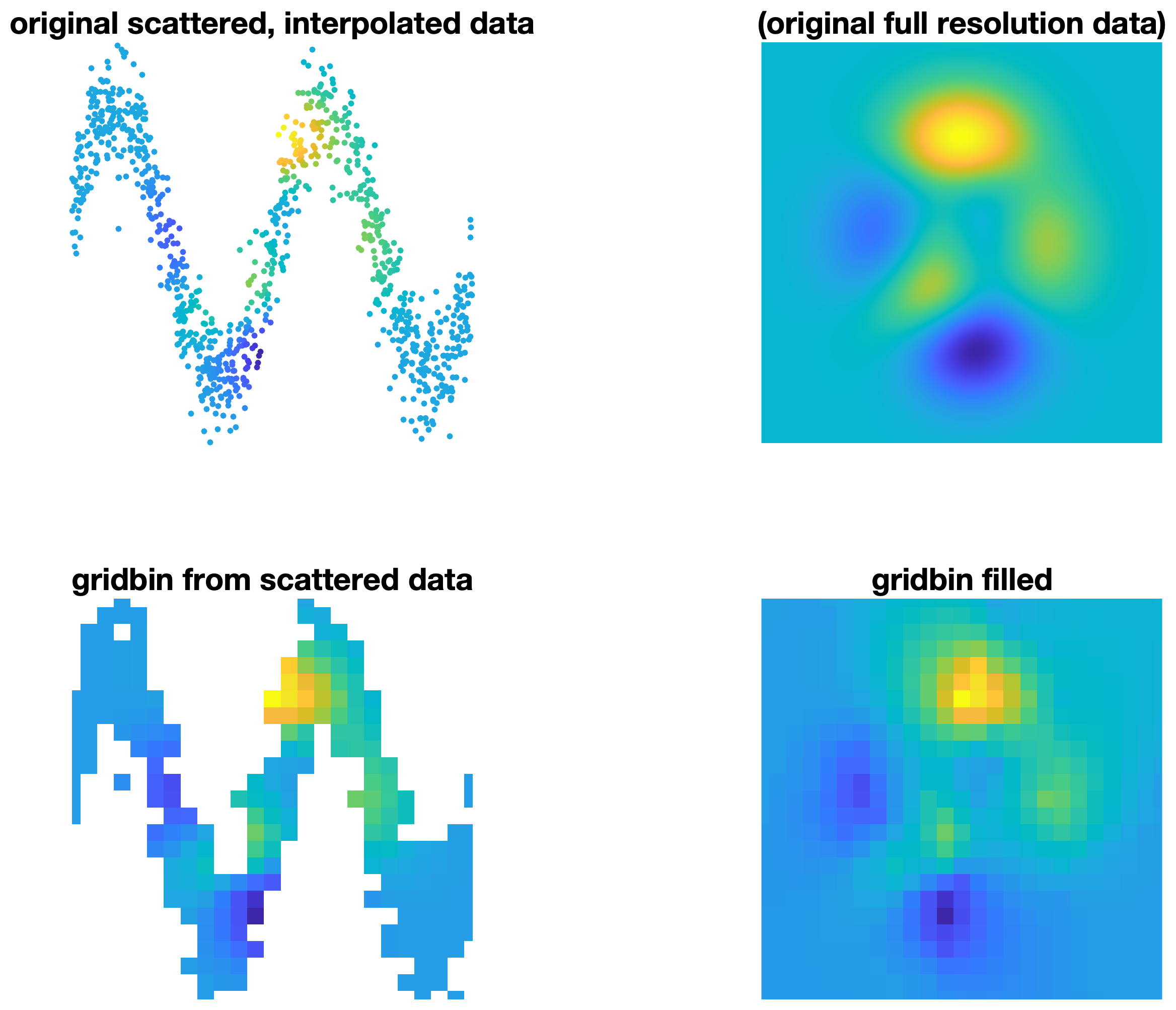## gridbin

version 1.0.0 (533 KB) by
A MATLAB function for gridding large datasets of scattered data.

Updated 02 Sep 2021

From GitHub# gridbin

A fast MATLAB function for gridding very large datasets of scattered data. This function is somewhat similar to Matlab's built-in `griddata` or John D'Errico's `gridfit`, with the differences being that

1. `gridbin` returns the mean value of all scattered data points within every cell of a grid rather than interpolating or fitting a surface.
2. `gridbin` is much faster than `griddata` or `gridfit`.
3. `gridbin` can handle much larger scattered datasets than `griddata` or `gridfit`.
4. `gridbin` can return statistics. For example, `gridbin` can return the standard deviation of all scattered data points within a grid cell simply by adding `@std` to the function call.

## Syntax

`````` vq = gridbin(x,y,v,xq,yq)
vq = gridbin(...,@func)
[vq,N] = gridbin(...)
``````

## Description

`vq = gridbin(x,y,v,xq,yq)` produces a 2D grid of values vq at the coordinates xq,yq. Inputs x,y,v may be scattered data, and the output vq is the mean of all scattered values v within each spatial bin.

`vq = gridbin(...,@func)` applies any function @func to the v data. By default, @func is @mean, meaning that vq contains the mean of all values v within each spatial bin.

`[vq,N] = gridbin(...)` also returns a grid N containing the number of observations v in each bin.### Cite As

##### MATLAB Release Compatibility
Created with R2021a
Compatible with any release
##### Platform Compatibility
Windows macOS Linux